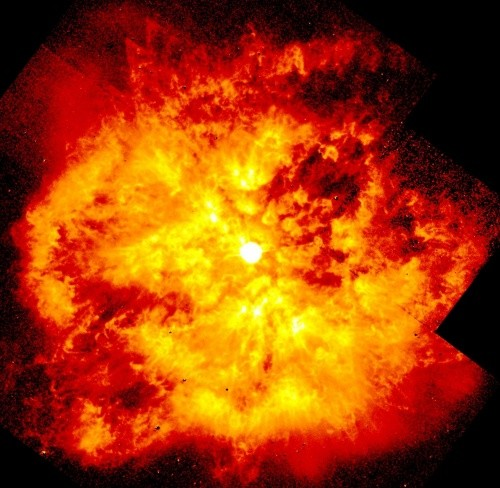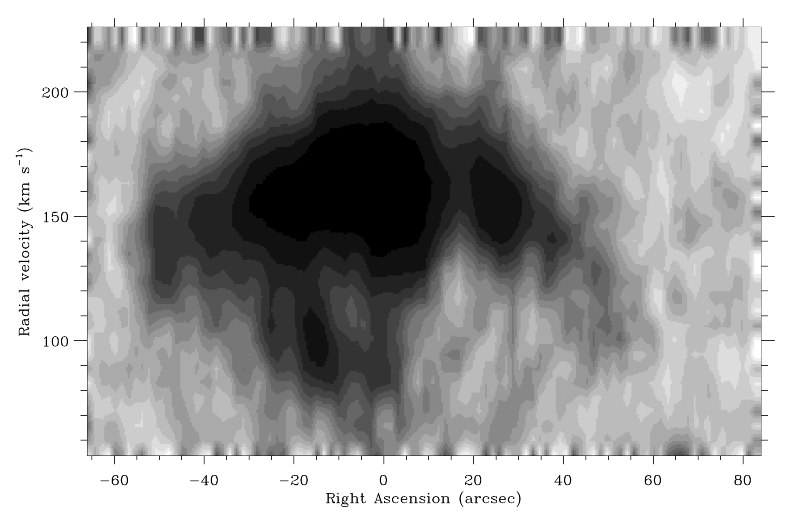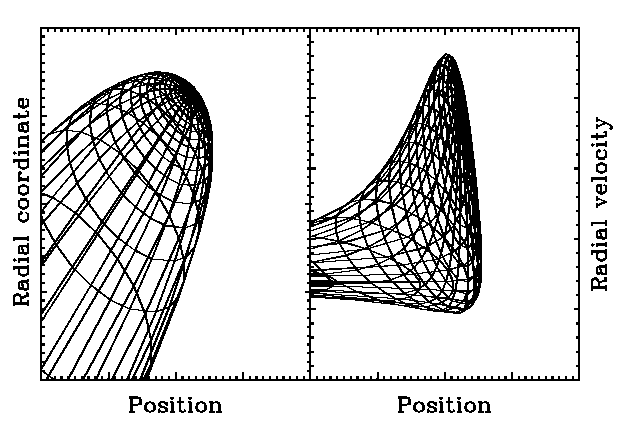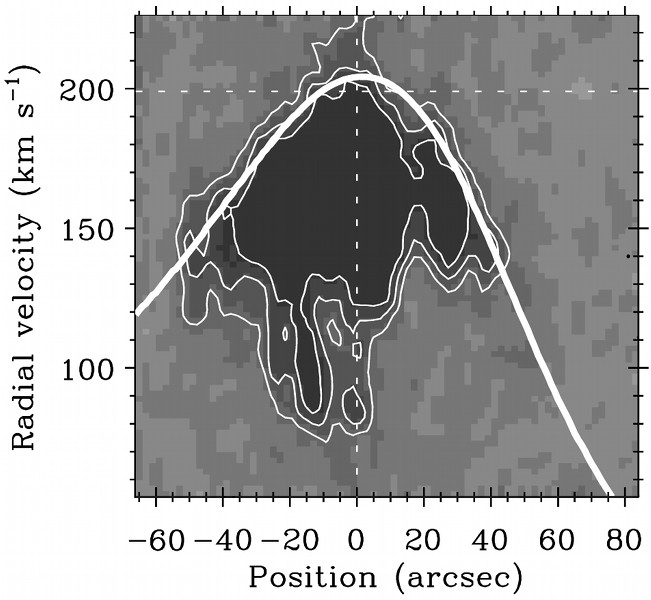# The dynamics of the ring nebula M1-67 around WR124Figure 1.  HST image of M1-67.
M1-67 is a nebular structure around the Wolf-Rayet star WR 124. The star is of spectral type WN8, which indicates that the star has probably just recently entered the Wolf-Rayet phase. The nebula around it looks very unstructured and clumpy (see Figure 1), but in the same time, (parts of) rings and arcs are clearly visible. These indicate that the nebula is probably caused by a number of discrete outbursts, possibly during a Luminous Blue Variable phase. The goal of this project was to find the number, geometries and dynamical timescales of these outbursts.Figure 2.  Emission distribution of M1-67 in the RA-v plane.

We found that the situation was more complicated than we had thought at first. It turns out that the star is moving with a velocity of about 180 km s-1 with respect to the local Interstellar Medium (ISM). In stead of a nice wind-blown bubble with a radius of about 30 pc, the star is surrounded by a bow shock and is just 1.3 pc away from the front of this bow shock. Because the star is moving away from us at an angle of less than 30° from the line of sight we do not see this bow shock in Figure 1, but it is visible from radial-velocity observations. In Figure 2, the emission distribution in the Right Ascension - Radial velocity plane is displayed, derived from Fabry-Pérot observations by Grosdidier et al.  The star has a radial velocity of about 199 km s-1 and sits at 0" in this image.Figure 3.  A 3D bow shock model for i=50°, PA=45°. Left panel: position-position plot, right panel: position-velocity plot.

To model this bow shock, we used simple analytical expressions derived by Canto, Raga and Wilkin.  They give the geometry and velocity along the surface of a 2D bow shock. From this, we created a 3D model, that can be rotated to any orientation. After that, the radial coordinate is converted to a radial velocity, so that we have two spatial coordinates and a radial velocity coordinate, that can be compared to the observational data like in Figure 2. An example of such a 3D bow shock model, in the RA - radial coordinate plane and RA - velocity plane, is shown in Figure 3.Figure 4.  Position-velocity plot of the model output (thick line) plotted over the data (grey scales and thin contours).

To obtain likely values for the star-ISM velocity, inclination and position angle, we used models of galactic rotation to obtain the ISM velocity and subtracted the known velocity of WR 124. Together with the observed stellar mass loss and terminal wind velocity and an assumed ISM density, these quantities define the geometry and orientation of the bow shock. We did this for different distances and different possible proper motions (there is a large error in the proper motion of WR 124, because of its large distance of about 6.5 kpc) and found that the model output resembles the data remarkably well, as shown in Figure 4. The thick line represents a contour around the model output mesh as shown in the right panel of Figure 3. The underlying grey-scaled image and its thin contours are the data from Figure 2.

This study was my undergraduate project and was published in Van der Sluys and Lamers, 2003.

References:
  Grosdidier, Y., et al., 1999, Proc. IAU Symp. 193, 356
  Canto, J., Raga, A.C. & Wilkin, F.P., 1996, ApJ 469, 729
  Nugis, T. & Lamers, H.J.G.L.M., 2000, A&A 360, 227